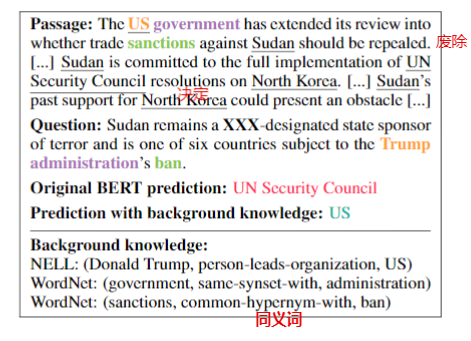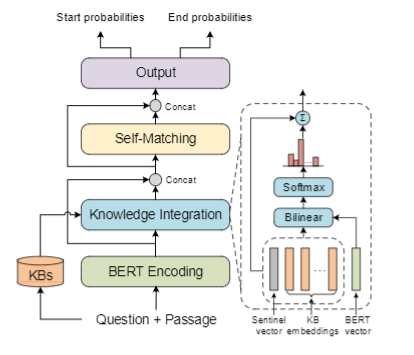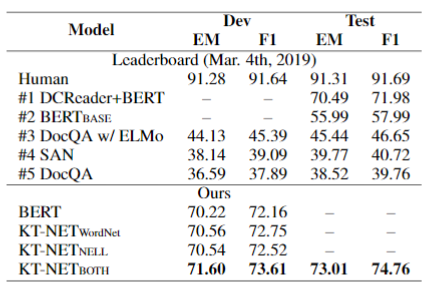# Enhancing Pre-Trained Language Representations with Rich Knowledge for Machine Reading Comprehension

## Introduction## Approach

KT-NET主要有四个模块：BERT encoding layer负责获取问题和段落的上下文表示；knowledge integration layerKnowledge Embedding and Retrieval

BERT Encoding Layer 首先将段落P和问题Q拼接成 $S=[\langle\mathrm{CLS}\rangle, Q,\langle\mathrm{SEP}\rangle, P,\langle\mathrm{SEP}\rangle]$，对S中的每一个token，使用 token, position, segment 编码 $\mathbf{h}_{i}^{0}=\mathbf{s}_{i}^{\mathrm{tok}}+\mathbf{s}_{i}^{\mathrm{pos}}+\mathbf{s}_{i}^{\mathrm{seg}}$，Q中的所有词认为是同一个segment，共享相同的segment embedding，而P中的所有词则共享另一个segment embedding，然后通过Transformer encoders，最终得到 $\left\{\mathbf{h}_{i}^{L}\right\}_{i=1}^{m+n+3} \in \mathbb{R}^{d_{1}}$。

Knowledge Integration Layer 这个模块负责将上下文信息与KB知识相融合，是整个模型的核心。本质上利用BERT表示$\mathbf{h}_{i}^{L} \in \mathbb{R}^{d_{1}}$对KB concepts候选集$C(s_{i})$做注意力，来选取最相关的KB concepts。作者使用 $\alpha_{i j} \propto \exp \left(\mathbf{c}_{j}^{\top} \mathbf{W h}_{i}^{L}\right)$ 计算注意力权重。因为KB concepts不一定与token密切相关，作者采取了类似Leveraging knowledge bases in lstms for improving machine reading的方法，引入了一个 knowledge sentinel $\overline{\mathbf{c}} \in \mathbb{R}^{d_{2}}$，同样计算相似度：$\beta_{i} \propto \exp \left(\mathbf{\overline{c}}^{\top} \mathbf{W} \mathbf{h}_{i}^{L}\right)$，然后：
$$\mathbf{k}_{i}=\sum_{j} \alpha_{i j} \mathbf{c}_{j}+\beta_{i} \overline{\mathbf{c}}$$

Self-Matching Layer 本质上是一个自注意力层，目的是构建context embedding $\mathbf{h}_{i}^{L}$ 与 knowledge embedding $\mathbf{k}_{i}$ 之间的交互。作者构建了两层交互：direct 和 indirect。首先通过 $r_{i j}=\mathbf{w}^{\top}\left[\mathbf{u}_{i}, \mathbf{u}_{j}, \mathbf{u}_{i} \odot \mathbf{u}_{j}\right]$ 得到相似度矩阵$\textbf{R}$ 以及按行softmax归一化后的权重矩阵$\textbf{A}$。直接交互是指$\mathbf{v}_{i}=\sum_{j} a_{i j} \mathbf{u}_{j}$，而间接交互是指：
\begin{aligned} \mathbf{\overline{A}} &=\mathbf{A}^{2} \\ \overline{\mathbf{v}}_{i} &=\sum_{j} \bar{a}_{i j} \mathbf{u}_{j} \end{aligned}

Output Layer 输出层与原始的NERT保持一致，只是简单的线性层，分别预测左右边界。
$$p_{i}^{1}=\frac{\exp \left(\mathbf{w}_{1}^{\top} \mathbf{o}_{i}\right)}{\sum_{j} \exp \left(\mathbf{w}_{1}^{\top} \mathbf{o}_{j}\right)}, \quad p_{i}^{2}=\frac{\exp \left(\mathbf{w}_{2}^{\top} \mathbf{o}_{i}\right)}{\sum_{j} \exp \left(\mathbf{w}_{2}^{\top} \mathbf{o}_{j}\right)}$$

## Experiments